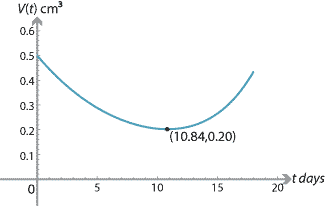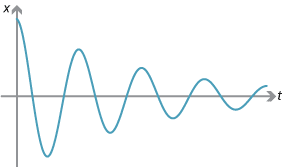## History and applications

### History

The history of calculus is discussed in the modules:

### Applications

#### Example: Chemotherapy

Malignant tumours respond to radiation therapy and chemotherapy. Consider a medical experiment in which mice with tumours are given a chemotherapeutic drug. At the time of the drug being administered, the average tumour size is about 0.5 cm$$^3$$. The tumour volume $$V(t)$$ after $$t$$ days is modelled by

$V(t) = 0.005e^{0.24t} + 0.495e^{-0.12t},$

for $$0\leq t \leq 18$$. Let us find the minimum point. The first and second derivatives are

\begin{align*} V'(t) &= 0.0012e^{0.24t} - 0.0594e^{-0.12t} \\ V''(t) &= 0.000288e^{0.24t} + 0.007128e^{-0.12t}. \end{align*}

Note that $$V''(t) > 0$$, for all $$t$$ in the domain. By solving $$V'(t) = 0$$, we find that the minimum point occurs when $$t \approx 10.84$$ days. The volume of the tumour is approximately 0.20 cm$$^3$$ at this time.#### Applications in physics

Calculus is essential in many areas of physics. Some additional applications of calculus to physics are given in the modules Motion in a straight line and The calculus of trigonometric functions.

#### Example: Damped simple harmonic motion

The displacement of a body of mass $$m$$ undergoing damped harmonic motion is given by the formula

$x = Ae^{-\frac{bt}{2m}}\cos(\omega t),$

where

$\omega = \sqrt{\dfrac{k}{m}-\Bigl(\dfrac{b}{2m}\Bigr)^2}.$

Here $$k$$ and $$b$$ are positive constants. (Note that damped simple harmonic motion reduces to simple harmonic motion when $$b=0$$.)The motion can be investigated by using calculus to find the turning points.

Since $$x = Ae^{-\frac{bt}{2m}}\cos(\omega t)$$, we have \begin{align*} \dfrac{dx}{dt} &= -\dfrac{b}{2m}Ae^{-\frac{bt}{2m}}\cos(\omega t) - A\omega e^{-\frac{bt}{2m}}\sin(\omega t) \\ &= -Ae^{-\frac{bt}{2m}}\Bigl(\dfrac{b}{2m}\cos(\omega t) + \omega\sin(\omega t)\Bigr). \end{align*} The stationary points are found by putting $$\dfrac{dx}{dt} = 0$$. This implies $\tan(\omega t) = -\dfrac{b}{2m\omega}.$

Here is example from optics using related rates of change.

#### Example: Thin lens formula

The thin lens formula in physics is

$\dfrac{1}{s} + \dfrac{1}{S} = \dfrac{1}{f},$

where $$s$$ is the distance of an object from the lens, $$S$$ is the distance of the image from the lens, and $$f$$ is the focal length of the lens. Here $$f$$ is a constant, and $$s$$ and $$S$$ are variables.

Suppose the object is moving away from the lens at a rate of 3 cm/s. How fast and in which direction will the image be moving?

We are given $$\dfrac{ds}{dt} = 3$$. The question is: What is $$\dfrac{dS}{dt}$$?

We know by the chain rule that

$\dfrac{dS}{dt} = \dfrac{dS}{ds} \times \dfrac{ds}{dt} = \dfrac{dS}{ds} \times 3.$

Making $$S$$ the subject of the thin lens formula, we have $$S = \dfrac{fs}{s-f}$$. The derivative of $$S$$ with respect to $$s$$ is

$\dfrac{dS}{ds} = \dfrac{-f^2}{(s-f)^2}.$

Thus

$\dfrac{dS}{dt} = \dfrac{-f^2}{(s-f)^2} \times 3 = \dfrac{-3f^2}{(s-f)^2}.$

The image is moving towards the lens at $$\dfrac{3f^2}{(s-f)^2}$$ cm/s.

Next page - Answers to exercises